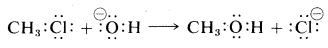# 8.1: Prelude to Nucleophilic Substitution and Elimination Reactions

$$\newcommand{\vecs}{\overset { \rightharpoonup} {\mathbf{#1}} }$$ $$\newcommand{\vecd}{\overset{-\!-\!\rightharpoonup}{\vphantom{a}\smash {#1}}}$$$$\newcommand{\id}{\mathrm{id}}$$ $$\newcommand{\Span}{\mathrm{span}}$$ $$\newcommand{\kernel}{\mathrm{null}\,}$$ $$\newcommand{\range}{\mathrm{range}\,}$$ $$\newcommand{\RealPart}{\mathrm{Re}}$$ $$\newcommand{\ImaginaryPart}{\mathrm{Im}}$$ $$\newcommand{\Argument}{\mathrm{Arg}}$$ $$\newcommand{\norm}{\| #1 \|}$$ $$\newcommand{\inner}{\langle #1, #2 \rangle}$$ $$\newcommand{\Span}{\mathrm{span}}$$ $$\newcommand{\id}{\mathrm{id}}$$ $$\newcommand{\Span}{\mathrm{span}}$$ $$\newcommand{\kernel}{\mathrm{null}\,}$$ $$\newcommand{\range}{\mathrm{range}\,}$$ $$\newcommand{\RealPart}{\mathrm{Re}}$$ $$\newcommand{\ImaginaryPart}{\mathrm{Im}}$$ $$\newcommand{\Argument}{\mathrm{Arg}}$$ $$\newcommand{\norm}{\| #1 \|}$$ $$\newcommand{\inner}{\langle #1, #2 \rangle}$$ $$\newcommand{\Span}{\mathrm{span}}$$$$\newcommand{\AA}{\unicode[.8,0]{x212B}}$$

Substitution reactions involve the replacement of one atom or group $$\left( \ce{X} \right)$$ by another $$\left( \ce{Y} \right)$$:

$\ce{RX} + \ce{Y} \rightarrow \ce{RY} + \ce{X}$

We already have described one very important type of substitution reaction, the halogenation of alkanes (Section 4-4), in which a hydrogen atom is replaced by a halogen atom ($$\ce{X} = \ce{H}$$, $$\ce{Y} =$$ halogen). The chlorination of 2,2-dimethylpropane is an example:Reactions of this type proceed by radical-chain mechanisms in which the bonds are broken and formed by atoms or radicals as reactive intermediates. This mode of bond-breaking, in which one electron goes with $$\ce{R}$$ and the other with $$\ce{X}$$, is called homolytic bond cleavage:There are a large number of reactions, usually occurring in solution, that do not involve atoms or radicals but rather involve ions. They occur by heterolytic cleavage as opposed to homolytic cleavage of electron-pair bonds. In heterolytic bond cleavage, the electron pair can be considered to go with one or the other of the groups $$\ce{R}$$ and $$\ce{X}$$ when the bond is broken. As one example, $$\ce{Y}$$ is a group such that it has an unshared electron pair and also is a negative ion. A heterolytic substitution reaction in which the $$\ce{R} \colon \ce{X}$$ bonding pair goes with $$\ce{X}$$ would lead to $$\ce{RY}$$ and $$\colon \ce{X}^\ominus$$,A specific substitution reaction of this type is that of chloromethane with hydroxide ion to form methanol:In this chapter, we shall discuss substitution reactions that proceed by ionic or polar mechanisms in which the bonds cleave heterolytically. We also will discuss the mechanistically related elimination reactions that result in the formation of carbon-carbon multiple bonds:These reactions often are influenced profoundly by seemingly minor variations in the structure of the reactants, in the solvent, or in the temperature. It is our purpose to show how these reactions can be understood and how they can be used to prepare other useful organic compounds. But first it will be helpful to introduce the concepts of nucleophilic and electrophilic reagents, and to consider the $$\Delta H$$ values for heterolytic bond breaking.